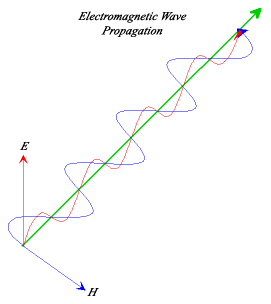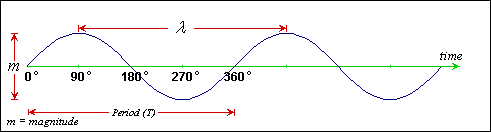Fundamentals of Light & Optics

Wave Theory

Light exhibits properties of both waves and particles, and is referred to as wave-particle duality. Generally, light behaves like as wave. Lets take a closer look at waves;Fig 1.2 - The Electromagnetic Wave

Light waves are composed of both an oscillating electrical (E) field and magnetic (H) field, together they are known as electromagnetic waves - i.e., light is an electromagnetic wave.

Electromagnetic fields oscillate perpendicular (90°) to the direction of wave travel, and perpendicular to each other. Light waves also transverse waves as they oscillate in the direction traverse to the direction of wave travel (as opposed to sound waves, which are longitudinal waves).

The sinusoidal wave is a fundamental (most basic) waveform and commonly found in nature. When dealing with light waves, we refer to the sine wave. Lets take a look at the sine wave in more detail;Fig 1.3 - The Sine Wave

Waves have three important characteristics:
• Wavelength
• Period / Frequency
• Amplitude (Magnitude)

Referring to the diagram of the sine wave;

The wavelength of the wave is calculated by measuring the distance between one positive (or negative) peak and the next positive (or negative) peak.

The period (T) of the waveform is the time to complete one full cycle, - i.e, one 0 to 360° sweep. The relationship of frequency and the period is given by the equation:

f = 1 / T

T = 1 / f

As an example, 2Hz has a period of 0.5 sec, 2kHz a period of 0.05 msec.

The waveforms are always in the time domain and go on for infinity.

Wavelength: - This is the distance between peaks of a wave. Wavelengths are measured in units of length - meters, When dealing with light, wavelengths are in the order of nanometres (1 x 10-9).

Frequency: - This is the number of peaks that will travel past a point in one second. Frequency is measured in cycles per second. The term given to this is Hertz (Hz) named after the 19th century discoverer of radio waves - Heinrich Hertz. 1 Hz = 1 cycle per second.

The speed of a wave, known as Phase Velocity, can be calculated using the following equation:

vp = λ / T

We are also able to calculate phase velocity by multiplying wavelength and frequency together. Phase velocity is measured in units of length (distance) per second:

Wavelength x Frequency = Velocity (Speed)

The amplitude of a wave relates to the size (magnitude) of the oscillations.

In the next section, we will cover particle theory in more detail.

This Document Complies to W3C XHTML 1.0 Strict Standards